## MP Board Class 6th Sanskrit Solutions सुरभिः

MP Board Class 6th Sanskrit Solutions Guide Pdf Free Download संस्कृत सुरभिः are part of MP Board Class 6th Solutions. Here we have given Madhya Pradesh Syllabus MP Board Surbhi Sanskrit Book Class 6 Solutions Pdf.

## MP Board Class 6th Sanskrit Book Solutions Surbhi

Here we have given MP Board Surbhi Samanya Sanskrit Book Class 6 Solutions Kaksha 6 (संस्कृत सामान्य सुरभिः कक्षा 6).

Surbhi Sanskrit Book Class 6 Solutions

पाठ का हिन्दी अनुवाद, शब्दार्थ एवं अभ्यास

MP Board Class 6th Sanskrit Model Question Paper (आदर्श प्रश्नपत्रम्)

We hope the given MP Board Class 6th Sanskrit Solutions Guide Pdf Free Download संस्कृत सुरभिः will help you. If you have any query regarding Madhya Pradesh Syllabus MP Board Class 6th Sanskrit Book Solutions Surbhi Pdf, drop a comment below and we will get back to you at the earliest.

## MP Board Class 6th Hindi Solutions सुगम भारती, भाषा भारती

MP Board Class 6th Hindi Solutions Guide Pdf Free Download of हिंदी सुगम भारती, भाषा भारती are part of MP Board Class 6th Solutions. Here we have given Madhya Pradesh Syllabus MP Board Class 6 Hindi Book Solutions Sugam Bharti, Bhasha Bharti Pdf Special and General.

## MP Board Class 6th Hindi Book Solutions Sugam Bharti

Here we have given MP Board Class 6 General Hindi Sugam Bharti Solutions Hindi Samanya Kaksha 6 (सुगम भारती हिंदी सामान्य कक्षा 6).

Sugam Bharti Hindi Book Class 6 Solutions

MP Board Class 6th General Hindi Model Question Paper

## MP Board Class 6th Hindi Book Solutions Bhasha Bharti

Here we have given MP Board Class 6 Special Hindi Bhasha Bharti Solutions Hindi Vishisht Kaksha 6 (भाषा भारती हिंदी विशिष्ट कक्षा 6).

Bhasha Bharti Hindi Book Class 6 Solutions

MP Board Class 6th Special Hindi Model Question Paper

We hope the given MP Board Class 6th Hindi Solutions Guide Pdf Free Download of हिंदी सुगम भारती, भाषा भारती will help you. If you have any query regarding Madhya Pradesh Syllabus MP Board Class 6 Hindi Book Solutions Sugam Bharti, Bhasha Bharti Pdf Special and General, drop a comment below and we will get back to you at the earliest.

## MP Board Class 6th Solutions

MP Board Class 6th Books Solutions Guide Pdf download all subjects in both Hindi Medium and English Medium are part of MP Board Solutions. Here we have given NCERT Madhya Pradesh Syllabus MP Board Class 6 Text Book Solutions Pdf.

## MP Board Class 6th Books Solutions

We hope the given MP Board Class 6th Books Solutions Guide Pdf download all subjects in both Hindi Medium and English Medium will help you. If you have any query regarding NCERT Madhya Pradesh Syllabus MP Board Class 6 Text Book Solutions Pdf, drop a comment below and we will get back to you at the earliest.

## MP Board Class 6th English Solutions General & Special Series

MP Board Class 6th English Reader Book Solutions Guide Pdf Free Download are part of MP Board Class 6th Solutions. Here we have given Madhya Pradesh Syllabus MP Board Class 6 English Book Solutions Pdf General and Special Series.

## MP Board Class 6th English Book Solutions

### MP Board English Reader Special Series Class 6 Solutions

Here we have given MP Board English Reader Special Series Class 6 Solutions and Answers Guide.

MP Board Class 6th Special English Grammar

### MP Board English Reader General Series Class 6 Solutions

Here we have given MP Board English Reader General Series Class 6 Solutions and Answers Guide.

We hope the given MP Board Class 6th English Reader Book Solutions Guide Pdf Free Download will help you. If you have any query regarding Madhya Pradesh Syllabus MP Board Class 6 English Book Solutions Pdf General and Special Series, drop a comment below and we will get back to you at the earliest.

## MP Board Class 6th Maths Solutions गणित

MP Board Class 6th Maths Solutions Guide Pdf Free Download गणित in both Hindi Medium and English Medium are part of MP Board Class 6th Solutions. Here we have given NCERT Madhya Pradesh Syllabus MP Board Class 6 Maths Book Solutions Ganit Pdf.

## MP Board Class 6th Maths Book Solutions in Hindi Medium

MP Board Class 6th Maths Chapter 1 अपनी संख्याओं की जानकारी

MP Board Class 6th Maths Chapter 2 पूर्ण संख्याएँ

MP Board Class 6th Maths Chapter 3 संख्याओं के साथ खेलना

MP Board Class 6th Maths Chapter 4 आधारभूत ज्यामितीय अवधारणाएँ

MP Board Class 6th Maths Chapter 5 प्रारंभिक आकारों को समझना

MP Board Class 6th Maths Chapter 6 पूर्णांक

MP Board Class 6th Maths Chapter 7 भिन्न

MP Board Class 6th Maths Chapter 8 दशमलव

MP Board Class 6th Maths Chapter 9 आँकड़ों का प्रबंधन

MP Board Class 6th Maths Chapter 10 क्षेत्रमिति

MP Board Class 6th Maths Chapter 11 बीजगणित

MP Board Class 6th Maths Chapter 12 अनुपात और समानुपात

MP Board Class 6th Maths Chapter 13 सममिति

MP Board Class 6th Maths Chapter 14 प्रायोगिक ज्यामिती

## MP Board Class 6th Maths Book Solutions in English Medium

MP Board Class 6th Maths Chapter 1 Knowing Our Numbers

MP Board Class 6th Maths Chapter 2 Whole Numbers

MP Board Class 6th Maths Chapter 3 Playing With Numbers

MP Board Class 6th Maths Chapter 4 Basic Geometrical Ideas

MP Board Class 6th Maths Chapter 5 Understanding Elementary Shapes

MP Board Class 6th Maths Chapter 6 Integers

MP Board Class 6th Maths Chapter 7 Fractions

MP Board Class 6th Maths Chapter 8 Decimals

MP Board Class 6th Maths Chapter 9 Data Handling

MP Board Class 6th Maths Chapter 10 Mensuration

MP Board Class 6th Maths Chapter 11 Algebra

MP Board Class 6th Maths Chapter 12 Ratio and Proportion

MP Board Class 6th Maths Chapter 13 Symmetry

MP Board Class 6th Maths Chapter 14 Practical Geometry

We hope the given MP Board Class 6th Maths Solutions Guide Pdf Free Download गणित in both Hindi Medium and English Medium will help you. If you have any query regarding NCERT Madhya Pradesh Syllabus MP Board Class 6 Maths Book Solutions Ganit Pdf, drop a comment below and we will get back to you at the earliest.

## MP Board Class 6th Science Solutions विज्ञान

MP Board Class 6th Science Solutions Guide Pdf Free Download विज्ञान in both Hindi Medium and English Medium are part of MP Board Class 6th Solutions. Here we have given NCERT Madhya Pradesh Syllabus MP Board Class 6 Science Book Solutions Vigyan Pdf.

## MP Board Class 6th Science Book Solutions in English Medium

We hope the given MP Board Class 6th Science Solutions Guide Pdf Free Download विज्ञान in both Hindi Medium and English Medium will help you. If you have any query regarding NCERT Madhya Pradesh Syllabus MP Board Class 6 Science Book Solutions Vigyan Pdf, drop a comment below and we will get back to you at the earliest.

## MP Board Class 6th Social Science Solutions सामाजिक विज्ञान

MP Board Class 6th Social Science Solutions Guide Pdf Free Download सामाजिक विज्ञान in both Hindi Medium and English Medium are part of MP Board Class 6th Solutions. Here we have given Madhya Pradesh Syllabus MP Board Class 6 Social Science Book Solutions Samajik Vigyan Pdf.

## MP Board Class 6th Social Science Book Solutions in Hindi Medium

MP Board Class 6th Social Science आदर्श प्रश्न-पत्र

## MP Board Class 6th Social Science Book Solutions in English Medium

We hope the given MP Board Class 6th Social Science Solutions Guide Pdf Free Download सामाजिक विज्ञान in both Hindi Medium and English Medium will help you. If you have any query regarding Madhya Pradesh Syllabus MP Board Class 6 Social Science Book Solutions Samajik Vigyan Pdf, drop a comment below and we will get back to you at the earliest.

## MP Board Class 6th Maths Solutions Chapter 12 Ratio and Proportion Ex 12.2

Question 1.
Determine if the following are in proportion.
(a) 15, 45, 40, 120
(b) 33, 121, 9, 96
(c) 24, 28, 36, 48
(d) 32, 48, 70, 210
(e) 4, 6, 8, 12
(f) 33, 44, 75, 100
Solution: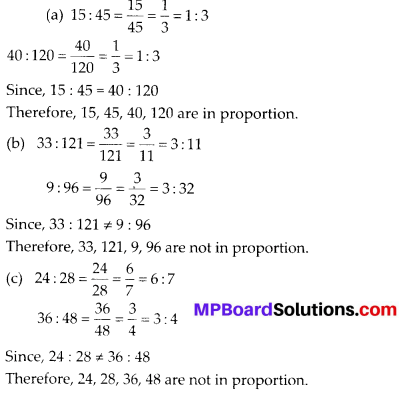Geometric Sequence Calculator is a free online tool that displays the geometric sequence for the given first term and the common ratio calculator.

Question 2.
Write True (T) or False (F) against each of the following statements:
(a) 16 : 24 :: 20 : 30
(b) 21 : 6 :: 35 : 10
(c) 12 : 18 :: 28 : 12
(d) 8 : 9 :: 24 : 27
(e) 5.2 : 3.9 :: 3 : 4
(f) 0.9 : 0.36 :: 10 : 4
Solution:
(a) True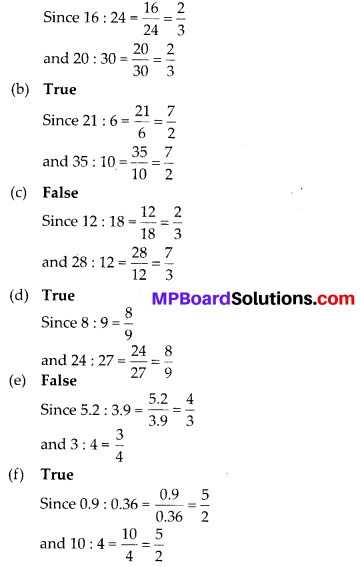Question 3.
Are the following statements true?
(a) 40 persons : 200 persons = Rs. 15 : Rs. 75
(b) 7.5 litres: 15 litres = 5 kg : 10 kg
(c) 99 kg : 45 kg = Rs. 44 : Rs. 20
(d) 32 m: 64 m = 6 sec : 12 sec
(e) 45 km : 60 km = 12 hours : 15 hours
Solution:
(a) 40 persons : 200 persons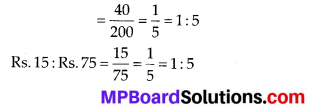∴ 40 persons : 200 persons = Rs. 15 : Rs. 75
Hence, the statement is true.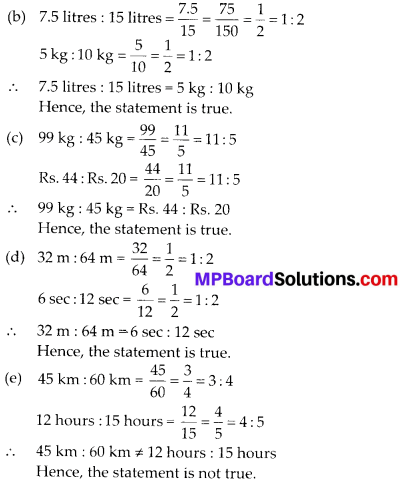Question 4.
Determine if the following ratios form a proportion. Also, write the middle terms and extreme terms where the ratios form a proportion.
(a) 25 cm : 1 m and Rs. 40: Rs. 160
(b) 39 litres: 65 litres and 6 bottles: 10 bottles
(c) 2 kg : 80 kg and 25 g : 625 g
(d) 200 ml: 2.5 litre and Rs. 4: Rs. 50
Solution:
(a) 25 cm : 1 m = 25 cm : (1 × 100) cmSince the ratios are equal, therefore these are in proportion.
Middle terms are 1 m and Rs. 40 and extreme terms are 25 cm and Rs. 160.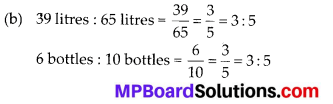Since the ratios are equal, therefore these are in proportion.
Middle terms are 65 litres and 6 bottles and extreme terms are 39 litres and 10 bottles.Since the ratios are not equal, therefore these are not in proportion.
(d) 200 ml : 2.5 litres = 200 ml: (2.5 × 1000) ml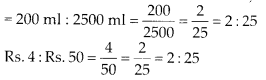Since the ratios are equal, therefore these are in proportion.
Middle terms are 2.5 litres and Rs. 4 and extreme terms are 200 ml and Rs. 50.

## MP Board Class 6th Maths Solutions Chapter 8 Decimals Ex 8.1

maths decimal rounding to the nearest hundredth ·

Question 1.
Write the following as numbers in the given table.Solution: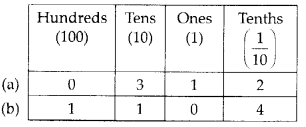When you round to 1 decimal place, you inspect the hundredths integer.

Question 2.
Write the following decimals in the place value table.
(a) 19.4
(b) 0.3
(c) 10.6
(d) 205.9
Solution: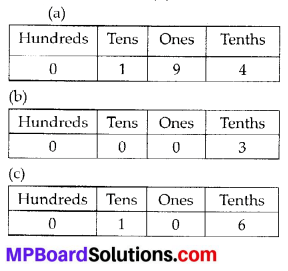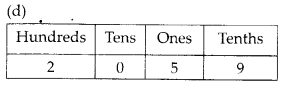What is 1/8 as a decimal? You can also take the fraction 3/4 and write it in highest terms to 75/100.

Question 3.
Write each of the following as decimals:
(a) Seven-tenths
(b) Two tens and nine-tenths
(c) Fourteen point six
(d) One hundred and two ones
(e) Six hundred point eight
Solution:
(a) Seven-tenths = 7 tenths = $$\frac{7}{10}$$ = 0.7
(b) Two tens and nine-tenths = $$2 \times 10+\frac{9}{10}$$
= 20 + 0.9 = 20.9
(c) Fourteen point six = 14.6
(d) One hundred and two-ones = 100 + 2 × 1
= 100 + 2 = 102
(e) Six hundred point eight = 600.8

Question 4.
Write each of the following as decimals: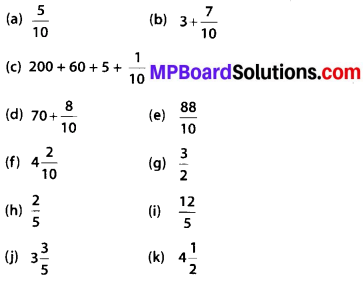Solution: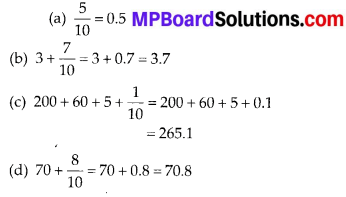To write 9/20 as a decimal you have to divide numerator by the denominator of the fraction.

Question 5.
Write the following decimals as fractions. Reduce the fractions to lowest form.
(a) 0.6
(b) 2.5
(c) 1.0
(d) 3.8
(e) 13.7
(f) 21.2
(g) 6.4
Solution: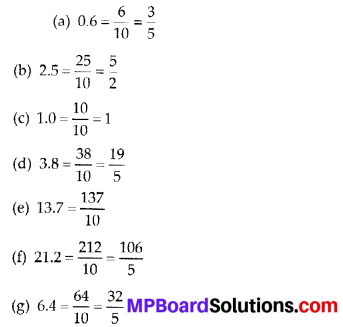Question 6.
Express the following as cm using decimals.
(a) 2 mm
(b) 30 mm
(c) 116 mm
(d) 4 cm 2 mm
(e) 162 mm
(f) 83 mm
Solution: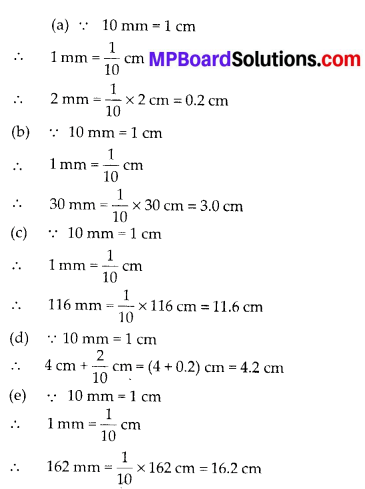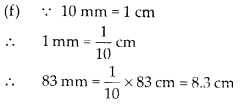Question 7.
Between which two whole numbers on the number line are the given numbers lie?
Which of these whole numbers is nearer the number?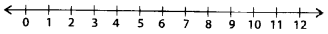Solution:
(a) 0.8
(b) 5.1
(C) 2.6
(d) 6.4
(e) 9.1
(f) 4.9
Solution:
(a) 0.8 lies between 0 and 1. From 0 to 1, 0.8 is nearer to 1
(b) 5.1 lies between 5 and 6. From 5 to 6, 5.1 is nearer to 5.
(c) 2.6 lies between 2 and 3. From 2 to 3, 2.6 is nearer to 3.
(d) 6.4 lies between 6 and 7. From 6 to 7, 6.4 is nearer to 6.
(e) 9.1 lies between 9 and 10. From 9 to 10, 9.1 is nearer to 9.
(f) 4.9 lies between 4 and 5. From 4 to 5, 4.9 is nearer to 5.Question 8.
Show the following numbers on the number line.
(a) 0.2
(b) 1.9
(c) 1.1
(d) 2.5
Solution:Question 9.
Write the decimal number represented by the points A, B, C, D on the given number line.Solution: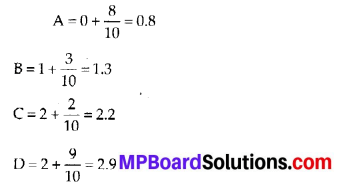Question 10.
(a) The length of Ramesh’s notebook is 9 cm 5 mm. What will be its length in cm?
(b) The length of a young gram plant is 65 mm. Express its length in cm.
Solution:
(a) 9 cm 5 mm = 9 cm + 5 mm
= $$\left(9+\frac{5}{10}\right)$$ cm = 9.5 cm

(b) 65 mm = $$\frac{65}{10}$$ cm = 6.5 cm

## MP Board Class 6th Maths Solutions Chapter 3 Playing With Numbers Ex 3.2

Question 1.
What is the sum of any two
(a) Odd numbers?
(b) Even numbers?
Solution:
(a) The sum of any two odd numbers is an even number.
As like, 1 + 3 = 4, 3 + 5 = 8

(b) The sum of any two even numbers is an even number.
As like, 2 + 4 = 6, 6 + 8 = 14The factor pair of 96 or any number as a set of two factors, which, when multiplied together, give a particular product.

Question 2.
State whether the following statements are True or False:
(a) The sum of three odd numbers is even.
(b) The sum of two odd numbers and one even number is even.
(c) The product of three odd numbers is odd.
(d) If an even number is divided by 2, the quotient is always odd.
(e) All prime numbers are odd.
(f) Prime numbers do not have any factors.
(g) Sum of two prime numbers is always even.
(h) 2 is the only even prime number.
(i) All even numbers are composite numbers.
(j) The product of two even numbers is always even.
Solution:
(a) False
Since, sum of two odd numbers is even and sum of one odd number and one even number is always odd.
(b) True
Since, sum of two odd numbers is even and sum of two even numbers is always even.
(c) True
(d) False
If an even number is divided by 2, then the quotient is either odd or even.
(e) False
Since, prime number 2 is even.
(f) False
Factors of prime numbers are 1 and the number itself.
(g) False
Sum of two prime numbers is either even or odd.
(h) True
(i) False
Since, even number 2 is prime i.e., not composite.
(j) True

Question 3.
The numbers 13 and 31 are prime numbers. Both these numbers have same digits 1 and 3. Find such pairs of prime numbers upto 100.
Solution:
Pairs of prime numbers having same digits upto 100 are 17 and 71; 37 and 73; 79 and 97So when we talk aqbout prime factorization of 38, we’re talking about the building blocks of the number.

Question 4.
Write down separately the prime and composite numbers less than 20.
Solution:
Prime numbers less than 20 are 2, 3, 5, 7, 11, 13, 17, 19
Composite numbers less than 20 are 4, 6, 8, 9, 10, 12, 14, 15, 16, 18

GCF Calculator is a free online tool that displays the Greatest Common Factor of two numbers given the inputs with detailed steps on how to approach.

Question 5.
What is the greatest prime number between 1 and 10?
Solution:
The greatest prime number between 1 and 10 is 7.Question 6.
Express the following as the sum of two odd primes.
(a) 44
(b) 36
(c) 24
(d) 18
Solution:
(a) 44 = 3 + 41
(b) 36 = 5 + 31
(c) 24 = 7 + 17
(d) 18 = 7 + 11

Question 7.
Give, three pairs of prime numbers whose difference is 2.
[Remark: Two prime numbers whose difference is 2 are called twin primes].
Solution:
Three pairs of prime numbers whose difference is 2 are 3 and 5; 5 and 7; 11 and 13.Question 8.
Which of the following numbers are prime?
(a) 23
(b) 51
(c) 37
(d) 26
Solution:
23 and 37 are prime numbers and 51 and 26 are composite numbers.
Thus, numbers in option (a) and (c) are prime.

Question 9.
Write seven consecutive composite numbers less than 100 so that there is no prime number between them.
Solution:
Seven consecutive composite numbers less than 100 are 90, 91, 92, 93, 94, 95, 96Question 10.
Express each of the following numbers as the sum of three odd primes:
(a) 21
(b) 31
(c) 53
(d) 61
Solution:
(a) 21 = 3 + 7 + 11
(b) 31 = 3 + 11 + 17
(c) 53 = 13 + 17 + 23
(d) 61 = 13 + 19 + 29Question 11.
Write five pairs of prime numbers less than 20 whose sum is divisible by 5.
(Hint :3 + 7 = 10)
Solution:
Since, 2 + 3 = 5; 7 + 13 = 20; 3 + 17 = 20; 2 + 13 = 15; 5 + 5 = 10 and 5, 10, 15, 20 all are
divisible by 5.
So, five pairs of prime numbers less than 20 whose sum is divisible by 5 are 2, 3; 2, 13; 3, 17; 7, 13; 5, 5.

Question 12.
Fill in the blanks:
(a) A number which has only two factors is called a ___.
(b) A number which has more than two factors is called a ___.
(c) 1 is neither ___ nor ___.
(d) The smallest prime number is ___.
(e) The smallest composite number is ___.
(f) The smallest even number is ___.
Solution:
(a) Prime number
(b) Composite number
(c) Prime number, composite number
(d) 2
(e) 4
(f) 2